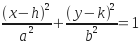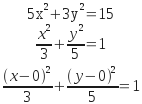SEARCH HOMEMath Central Quandaries & QueriesQuestion from Nae, a student: what is the ellipse center of 5x^2+3y^2=15Hi Nae.

The standard form of an ellipse iswhere (h, k) is the center and a and b control how much they extend horizontally and vertically.

So I think you can see that for your equation that h and k must be zero:Hope this helps,
Stephen La Rocque.Math Central is supported by the University of Regina and The Pacific Institute for the Mathematical Sciences.